2023-02-2300

## 目录

1. 认识排序算法
2. 冒泡算法（Bubble Sort）
3. 选择排序（Selection Sort）
4. 插入排序（Insertion Sort）
5. 归并排序（Merge Sort）
6. 快速排序（Quick Sort）
7. 堆排序（Heap Sort）

# 1. 认识排序算法

• 计算的时间复杂度：使用大 O 表示法，也可以实际测试消耗的时间；
• 内存使用量：比如外部排序，使用磁盘来存储排序的数据
• 稳定性：稳定排序算法会让原本相等键值的记录维持相对次序
• 排序的方法：插入、交换、选择、合并等等

1. 冒泡排序
2. 选择排序
3. 插入排序
4. 归并排序
5. 快速排序
6. 堆排序
7. ...

# 2. 冒泡算法（Bubble Sort）

1. 通过两两比较相邻的元素并交换它们的位置，从而使整个序列按照顺序排列
2. 一趟排序后，最大值总会移动到数组最后面，那么接下来就不用再考虑这个最大值了。
3. 一直重复这样的操作，最终就可以得到排序完成的数组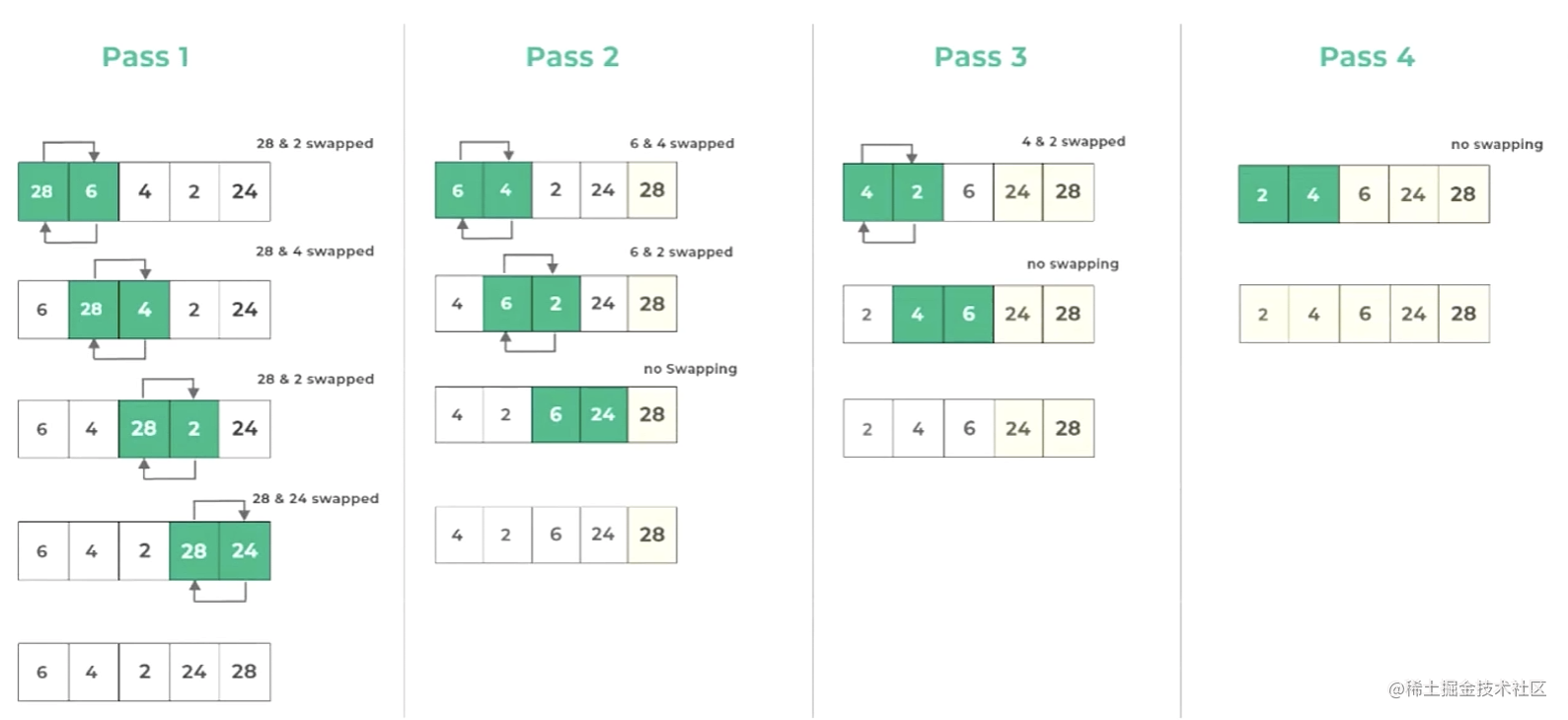```ts```function bubbleSort(arr: number[]): number[] {
const n = arr.length;
// 外层循环：0 ~ n-1
for (let i = 0; i < n; i++) {
// 内层循环找到最大值
for (let j = 1; j < n - i; j++) {
if (arr[j - 1] > arr[j]) {
// 交换两个数据
[arr[j - 1], arr[j]] = [arr[j], arr[j - 1]];
}
}
}
return arr;
}
``````

1. 时间复杂度：O(n2)
2. 空间复杂度：O(1)

1. 冒泡排序适用于数据规模较小的情况，因为它的时间复杂度为 O(n2)，对于大数据量的排序会变得很慢

2. 同时，它的实现简单，代码实现也容易理解，适用于学习排序算法的初学者

3. 但是，在实际的应用中，冒泡排序并不常用，因为它的效率很低

4. 因此，在实际应用中，冒泡排序通常被更高效的排序算法代替，如快速排序、归并排序

# 3. 选择排序（Selection Sort）

1. 首先在未排序的数列中找到最小（大）元素，然后将其存放到数列的起始位置
2. 接着，再从剩余未排序的元素中继续寻找最小（大）元素，然后放到已排序序列的末尾
3. 以此类推，直到所有元素均排序完毕

1. 如果某个元素位于正确的最终位置，则它不会被移动
2. 选择排序每次交换一对元素，它们当中至少有一个将被移到其最终位置上，因此对 `n` 个元素的表进行排序总共进行 至多 `n-1` 次交换
3. 在所有的完全依靠交换去移动元素的排序方法中，选择排序属于非常好的一种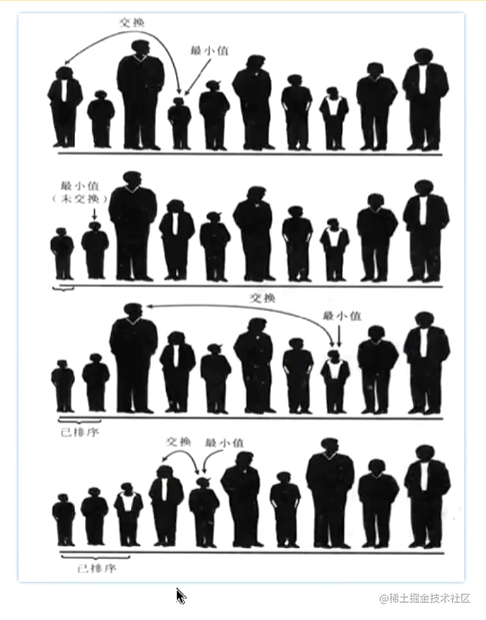```ts```function selectionSort(arr: number[]): number[] {
const n = arr.length;
// 外层循环作用：经过多少轮的找最小值
for (let i = 0; i < n; i++) {
let minIndex = i;
// 内存循环作用：每次找到最小值
for (let j = i + 1; j < n; j++) {
if (arr[j] < arr[minIndex]) {
minIndex = j;
}
}

if (minIndex !== i) {
[arr[minIndex], arr[i]] = [arr[i], arr[minIndex]];
}
}
return arr;
}
``````

1. 时间复杂度：O(n2)
2. 空间复杂度：O(1)

1. 虽然选择排序的实现非常简单，但是它的时间复杂度较高，对于大规模的数据排序效率较低
2. 总的来说，选择排序适用于小规模数据的排序和排序算法的入门学习，对于需要高效排序的场合，可以选择其他更为高效的排序算法

# 4. 插入排序（Insertion Sort）

1. 我们会将新牌和手牌中已有的牌进行比较，找一个合适的位置插入新牌
2. 如果新牌比某张牌小，那么我们就把这张牌向后移动一位，为新牌腾出位置
3. 一直比较直到找到一个合适的位置将新牌插入，这样就完成了一次插入操作。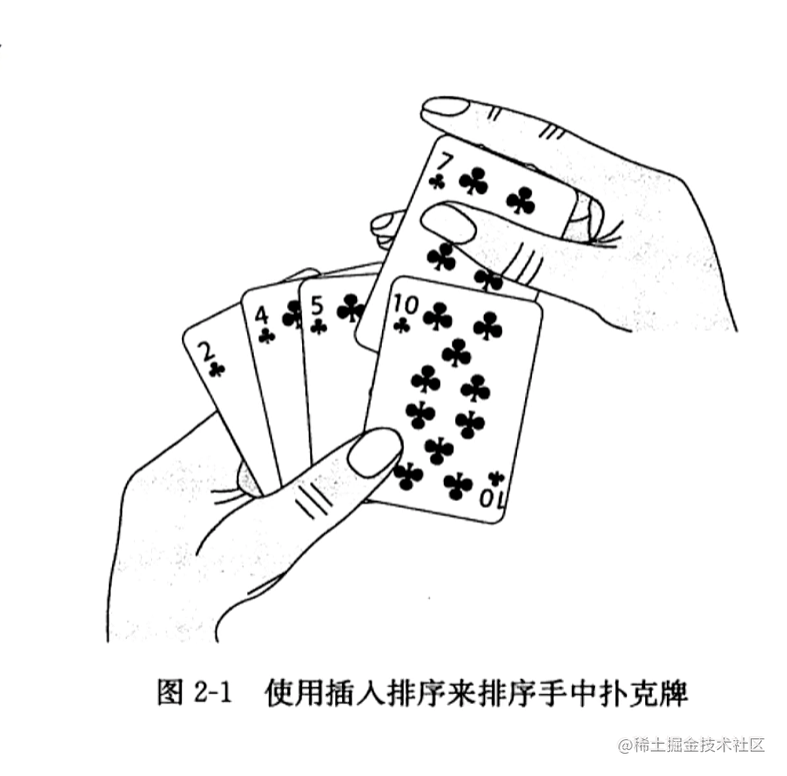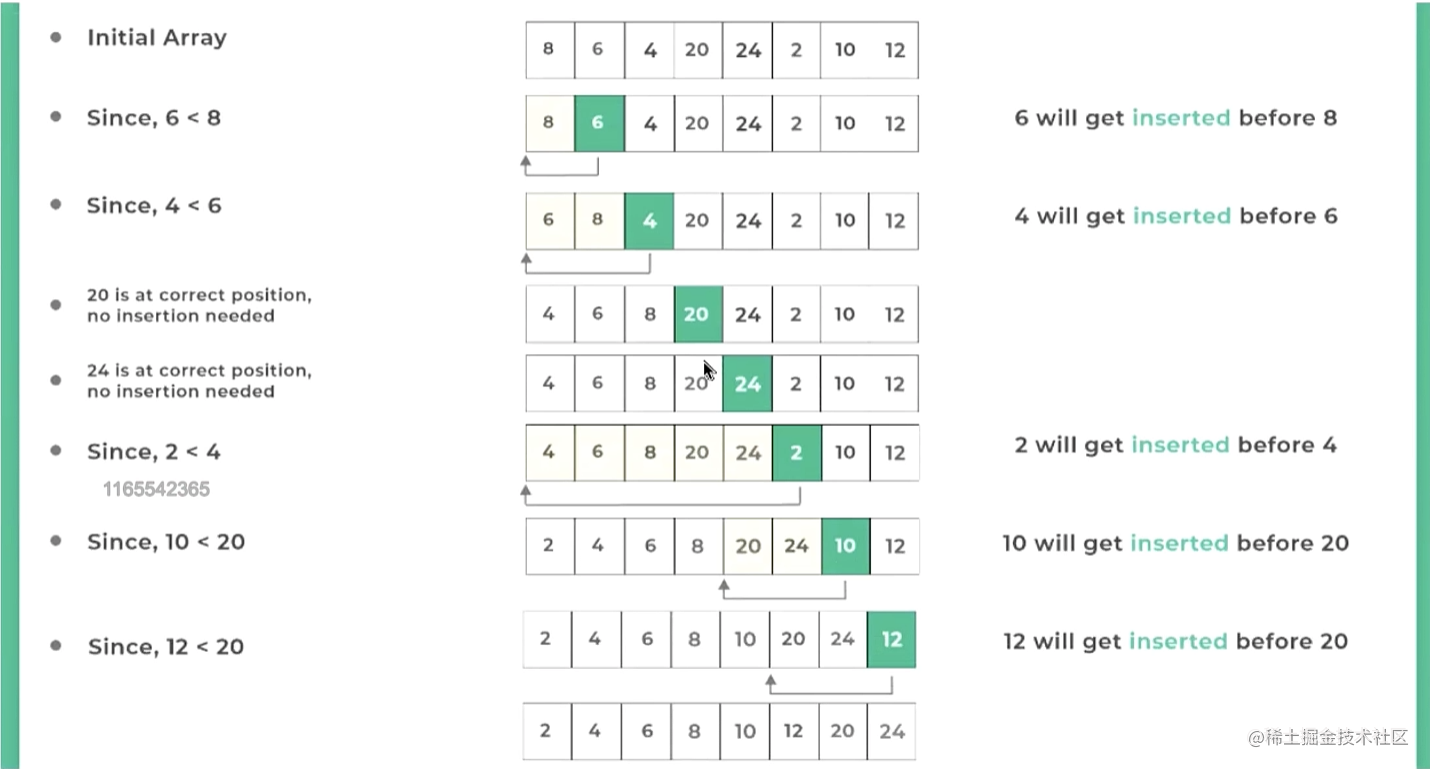```ts```function insertionSort(arr: number[]): number[] {
const n = arr.length;
for (let i = 1; i < n; i++) {
// 内层循环
const newNum = arr[i];
let j = i - 1;
while (arr[j] > newNum && j >= 0) {
arr[j + 1] = arr[j];
j--;
}
arr[j + 1] = newNum;
}
return arr;
}
``````

1. 时间复杂度：O(n2)
2. 空间复杂度：O(1)

1. 插入排序是一种简单直观的排序算法，它的基本思想就是将待排序数组分为已排序部分未排序部分，然后将未排序部分的每个元素插入到已排序部分的合适位置
2. 插入排序的时间复杂度为 O(n2)，虽然这个复杂度比较高，但是插入排序的实现非常简单，而且在某些情况下性能表现也很好（待排数组的带部分元素已经排序好）
3. 总之，插入排序虽然没有 快速排序归并排序 等高级排序算法的复杂性和高效性，但是它的实现非常简单，而且在一些特定的场景下表现也很好

# 5. 归并排序（Merge Sort）

1. 将待排序数组分成若干个子数组
2. 然后将相邻的子数组归并成一个有序数组
3. 最后再将这些有序数组归并（`merge`）成一个整体有序的数组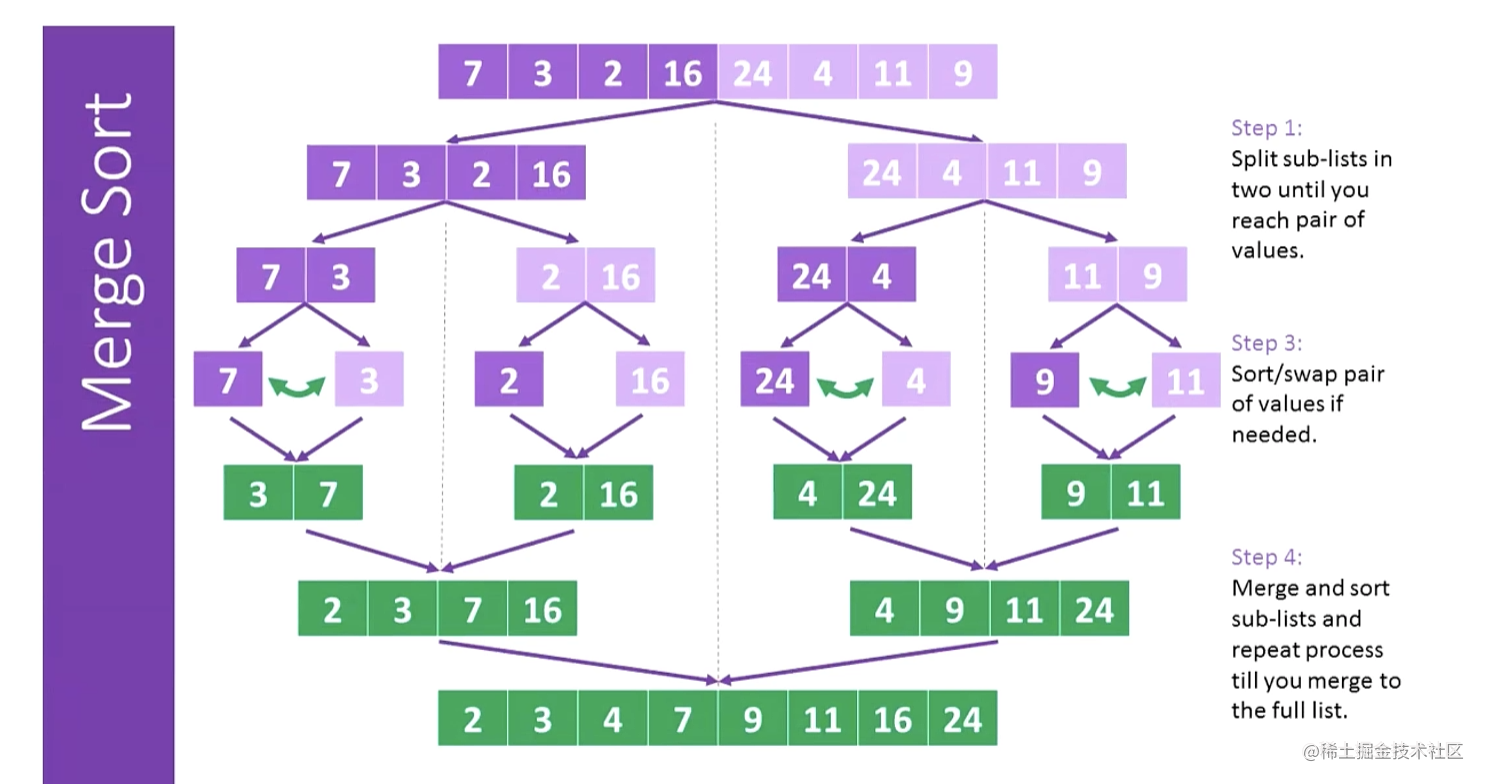```ts```const mergeSort = function (arr: number[]) {
if (arr.length === 1) return arr;

// 1. 分解(divide)：对数组进行分解（分解成链各个小数组）
// 1.1 递归地切割数组得到左边的数组left 和 右边的数组right
const mid = arr.length >> 1;
const left: number[] = mergeSort(arr.slice(0, mid));
const right: number[] = mergeSort(arr.slice(mid));

// 2. 合并（merge）：将两个子数组进行合并（双指针）
// 2.1 定义双指针
const result: number[] = [];
let leftIndex = 0;
let rightIndex = 0;
while (leftIndex < left.length && rightIndex < right.length) {
if (left[leftIndex] < right[rightIndex]) {
result[leftIndex + rightIndex] = left[leftIndex++];
} else {
result[leftIndex + rightIndex] = right[rightIndex++];
}
}

// 2.2 判断是否一个数组中海油剩余元素
// 循环完左边还有剩余
while (leftIndex < left.length) {
result[leftIndex + rightIndex] = left[leftIndex++];
}

// 循环完右边还有剩余
while (rightIndex < right.length) {
result[leftIndex + rightIndex] = right[rightIndex++];
}
return result;
};
``````

1. 时间复杂度：O(nlogn)
2. 空间复杂度：O(n)

1. 归并排序是一种非常高效的排序算法，它的核心思想是分治，即将待排序数组分成若干个子数组，分别对这些子数组进行排序，最后将排好序的子数组合并成一个有序数组。
2. 归并排序的时间复杂度为O(nlogn)
3. 虽然归并排序看起来比较复杂，但是只要理解了基本思路，实现起来并不困难，而且它是一种非常高效的排序算法。

# 6. 快速排序（Quick Sort）

1. 将一个大数组分成两个小数组，然后递归地对两个小数组进行排序
2. 具体实现方式是通过选择一个基准元素（pivot），将数组分成左右两部分左部分的元素都小于或等于基准元素，右部分的元素都大于基准元素
3. 然后，对左右两部分分别进行递归调用快速排序，最终将整个数组排序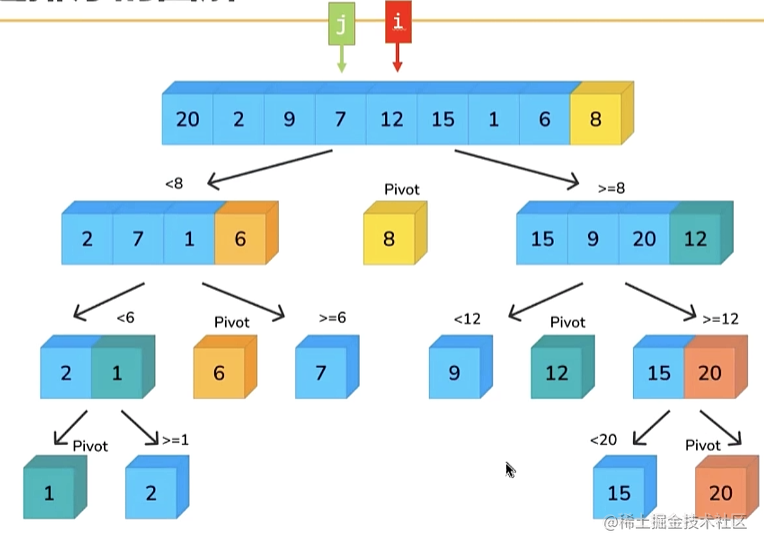```ts```const quickSort = function (arr: number[]): number[] {
partition(0, arr.length - 1);

function partition(left: number, right: number) {
if (left >= right) return;

// 1. 找到基准元素（pivot轴心）
const pivot = arr[right];

// 2. 双指针进行交换操作（左边都是比 pivot 小的数字，右边都是比 pivot 大的数字）
let i: number = left;
let j: number = right - 1;

while (i <= j) {
// 找到一个比 pivot 大的元素
while (arr[i] < pivot) {
i++;
}

// 找到一个比 pivot 小的元素
while (arr[j] > pivot) {
j--;
}

// 说明我们已经找到了 比pivot大的元素arr[i] 和 比pivot小的元素arr[j]
if (i <= j) {
[arr[i], arr[j]] = [arr[j], arr[i]];
i++;
j--;
}
console.log({ arr });
}
// 将 pivot 放在正确的位置
[arr[i], arr[right]] = [arr[right], arr[i]];

// 左右继续划分区域(partition)
partition(left, j);
partition(i + 1, right);
}

return arr;
};
``````

1. 快速排序的性能优于许多其他排序算法，因为它具有良好的局部性和使用原地排序的优点。
2. 快速排序是一种高效的排序算法，在实践中被广泛使用

# 7. 堆排序（Heap Sort）

1. 首先我们会构建一个最大堆
2. 然后，在我们将堆的根节点（也就是最大值）与堆的最后一个元素交换，这样最大值就被放在了正确的位置上。
3. 接着，我们将堆的大小减小一，并将剩余的元素重新构建成一个最大堆
4. 我们不断重复这个过程，直到堆的大小为 `1`
5. 这样，我们就得到了一个有序序列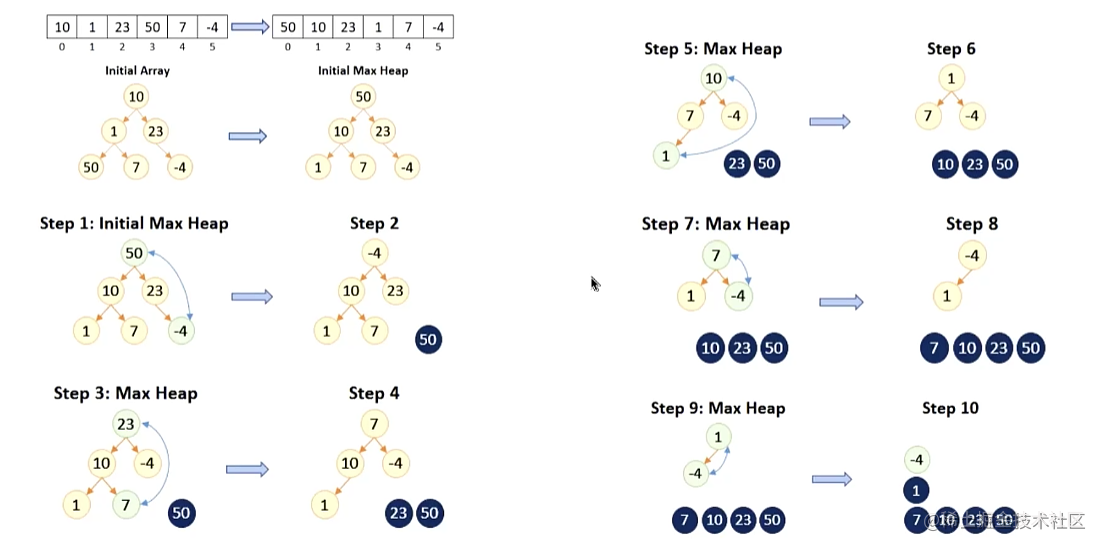```ts```function heapSort(arr: number[]): number[] {
// 1. 获取数组的长度
const n = arr.length;

// 2. 对 arr 进行原地建堆
// 2.1 从第一个非叶子节点开始进行下滤操作
const start = Math.floor(n / 2 - 1);
for (let i = start; i >= 0; i--) {
// 2.2 进行下滤操作
heapifyDown(arr, n, i);
}

// 3. 对最大堆进行排序
for (let i = n - 1; i > 0; i--) {
[arr, arr[i]] = [arr[i], arr];
heapifyDown(arr, i, 0);
}
return arr;
}

function heapifyDown(arr: number[], n: number, index: number) {
while (2 * index + 1 < n) {
// 1. 获取左右子节点的索引
const leftChildIndex = 2 * index + 1;
const rightChildIndex = 2 * index + 2;

// 2. 找出左右子节点较大的值
let largerIndex = leftChildIndex;
if (rightChildIndex < n && arr[rightChildIndex] > arr[leftChildIndex]) {
largerIndex = rightChildIndex;
}

// 3. 判断 index 位置的值比更大的子节点，直接 break
if (arr[index] >= arr[largerIndex]) {
break;
}

// 4. 和更大位置的进行交换
[arr[index], arr[largerIndex]] = [arr[largerIndex], arr[index]];
index = largerIndex;
}
}
``````

1. 堆的建立过程：堆的建立过程包括 `n/2` 次堆的向下调整操作，因此它的时间复杂度为 `O(n)`
2. 排序过程
1. 排序过程需要执行 `n` 次堆的删除的最大值操作，每次操作都需要将堆的最后一个元素与堆顶元素交换，然后向下调整堆
2. 每次向下调整操作的时间复杂度为 `O(logn)`，因此整个排序过程的时间复杂度为 `O(nlogn)`

1. 堆排序是一种高效的排序算法，它利用堆这种数据结构来实现排序
2. 堆排序具有时间复杂度 `O(logn)` 的优秀性能，并且由于它只使用了常数个辅助变量来存储堆的信息，因此空间复杂度为 `O(1)`
3. 但是，由于堆排序的过程是不稳定的，即相同元素的相对位置可能会发生变化，因此在某些情况下可能会导致排序结果不符合要求